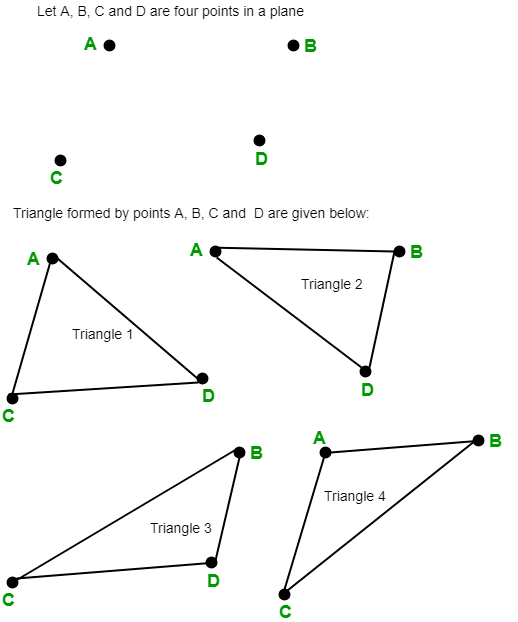# Number of triangles in a plane if no more than two points are collinear

Given n points in a plane and no more than two points are collinear, the task is to count the number of triangles in a given plane.

Examples:

Input :  n = 3
Output : 1

Input :  n = 4
Output : 4Let there are n points in a plane and no three or more points are collinear then number of triangles in the given plane is given by## C++

 // C++ program to find the number of  // triangles in a plane if no more  // then two points are collinear.  #include  using namespace std;     // Function to find number of triangles  // in a plane.  int countNumberOfTriangles(int n)  {         // Formula to find number of triangles      // nC3 = n * (n - 1) * (n - 2) / 6      return n * (n - 1) * (n - 2) / 6;  }     // Driver code  int main()  {      int n = 4;      cout << countNumberOfTriangles(n);      return 0;  }

## Java

 // Java program to find the number of  // triangles in a plane if no more  // then two points are collinear.  import java.io.*;     class GFG {         // Function to find number of triangles      // in a plane.      static int countNumberOfTriangles(int n)      {             // Formula to find number of triangle          // nC3 = n * (n - 1) * (n - 2) / 6          return n * (n - 1) * (n - 2) / 6;      }         // Driver code      public static void main(String[] args)      {          int n = 4;             System.out.println(              countNumberOfTriangles(n));      }  }

## Python3

 # Python3 program to find   # the number of triangles   # in a plane if no more  # then two points are collinear.     # Function to find number  # of triangles in a plane.  def countNumberOfTriangles(n) :             # Formula to find       # number of triangles      # nC3 = n * (n - 1) *      # (n - 2) / 6      return (n * (n - 1) *                  (n - 2) // 6)     # Driver Code  if __name__ == '__main__' :             n = 4     print(countNumberOfTriangles(n))                        # This code is contributed  # by ajit

## C#

 // C# program to find the   // number of triangles in   // a plane if no more then   // two points are collinear.  using System;     class GFG   {         // Function to find number      // of triangles in a plane.      static int countNumberOfTriangles(int n)      {             // Formula to find number           // of triangle           // nC3 = n * (n - 1) *          //           (n - 2) / 6          return n * (n - 1) *                      (n - 2) / 6;      }         // Driver code      public static void Main()      {          int n = 4;             Console.WriteLine(              countNumberOfTriangles(n));      }  }     // This code is contributed by anuj_67.

## PHP

 

Output:

4


My Personal Notes arrow_drop_upCheck out this Author's contributed articles.

If you like GeeksforGeeks and would like to contribute, you can also write an article using contribute.geeksforgeeks.org or mail your article to contribute@geeksforgeeks.org. See your article appearing on the GeeksforGeeks main page and help other Geeks.

Please Improve this article if you find anything incorrect by clicking on the "Improve Article" button below.

Improved By : vt_m, jit_t, pallav945